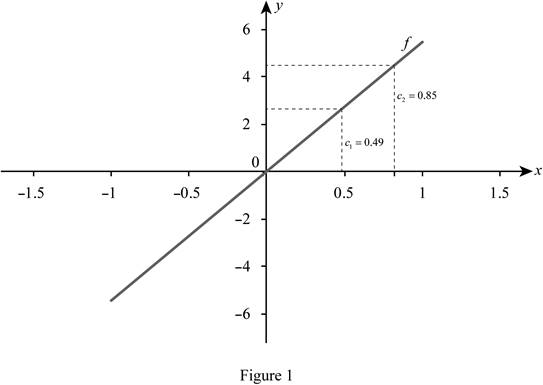# The average value of the function at the given interval.### Single Variable Calculus: Concepts...

4th Edition
James Stewart
Publisher: Cengage Learning
ISBN: 9781337687805### Single Variable Calculus: Concepts...

4th Edition
James Stewart
Publisher: Cengage Learning
ISBN: 9781337687805

#### Solutions

Chapter 6.5, Problem 10E

(a)

To determine

## To find: The average value of the function at the given interval.

Expert Solution

The average value of the function is 0.49.

### Explanation of Solution

Given information:

The function f(x) is f(x)=2xex2

f(x)=2xex2,[0,2].

Calculation:

The function is,

f(x)=2xex2 (1)

Find the average value of function fave on the interval.

fave=1baabf(x)dx (2)

Substitute 0 for a, 2 for b and 2xex2 for f(x) in Equation (2).

fave=120022xex2dx=02xex2dx (3)

Consider u=x2 (4)

Differentiate both side of the Equation (4).

du=2xdx

Substituteu for x2 and 12du for dx in Equation (3).

fave=02eu(12du)=1202eudu=12[eu]02 (5)

Substitute x2 for u in Equation (5).

fave=12(ex2)02=12(e22e0)=12(1e4)=0.49

Hence, the average value of the function is 0.49.

(b)

To determine

### To find: The value of c.

Expert Solution

The average value of c is 0.85.

### Explanation of Solution

Given information:

The function f(x) is f(x)=2xex2

f(x)=2xex2,[0,2].

Calculation:

Find the c as shown below.

f(c)=favg

Substitute c for x in Equation (1)

2cec2=0.49cec2=0.245 (6)

Take log on both sides in Equation (6).

c3=0.61c=0.85

Thus, the average value of c=0.85

(c)

To determine

Expert Solution

### Explanation of Solution

Draw the graph of f.

Sketch the graph of function f as shown in Figure 1.### Have a homework question?

Subscribe to bartleby learn! Ask subject matter experts 30 homework questions each month. Plus, you’ll have access to millions of step-by-step textbook answers!